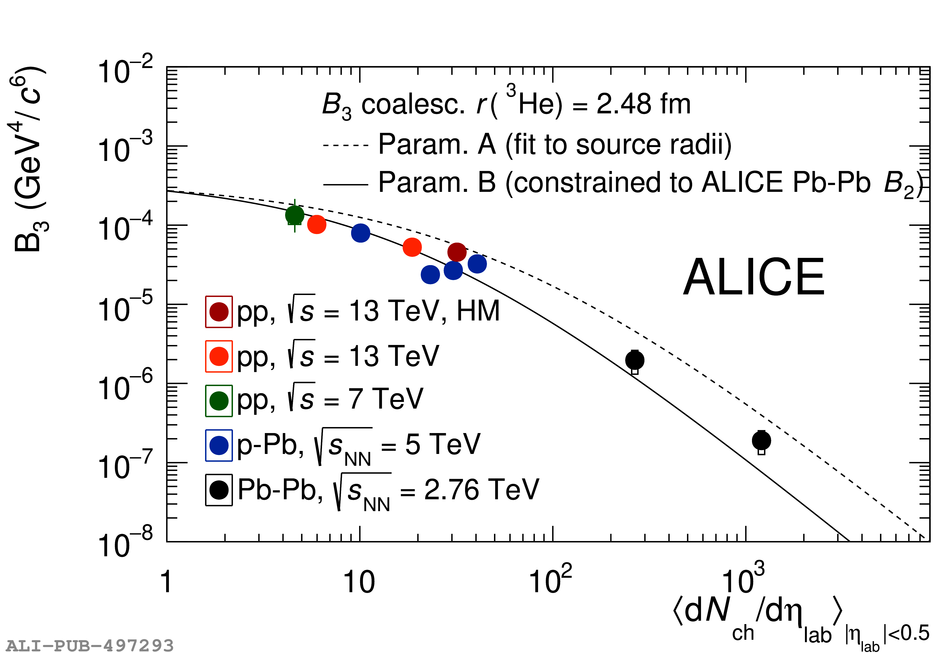# Figure 8

 (a): $B_2$ at $p_{\mathrm{T}}/A =$ 0.75~GeV/< em>c< /em> as a function of multiplicity in HM pp collisions at $\sqrt{s}~=~13$~TeV, in MB pp collisions at $\sqrt{s}~=~13$~TeV~ and at $\sqrt{s}~=~7$~TeV~, in p--Pb collisions at $\snn~=~5.02$~TeV~, and in Pb--Pb collisions at $\snn~=~2.76$~TeV~. (b): $B_3$ at $p_{\mathrm{T}}/A =$ 0.73~GeV$/$< em>c< /em> as a function of multiplicity in HM pp collisions at $\sqrt{s}~=~13$~TeV, in MB pp collisions at $\sqrt{s}~=~13$~TeV, in p--Pb collisions at $\snn~=~5.02$~TeV~, and in Pb--Pb collisions at $\snn~=~2.76$~TeV~. Vertical bars and boxes represent statistical and systematic uncertainties, respectively. The two lines are theoretical predictions based on two different parameterisations of the source radius.